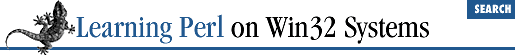home | O'Reilly's CD bookshelfs | FreeBSD | Linux | Cisco | Cisco Exam

### A.15 Chapter 16, System Information

1. Here's one way to do it.

```foreach \$host (@ARGV) {
print "\$host:\n";

print join(".", unpack("C4", \$a)), "\n";
}
}```

This code just takes a list of machine names, iterates over them, calling ``` get-hostbyname()``` for each one. We then enumerate each of the addresses, printing them out in dotted decimal notation.

2. Here's one way to do it:

```use

Win32::Registry;
\$p = shift || die "usage: \$0 path";
\$p =~ s#^\\##;
\$main::HKEY_LOCAL_MACHINE->Open(\$p, \$key) ||
die "Open: \$!";
\$key->GetValues(\%vals); # get values -hash ref
foreach \$k (keys %vals) {
\$key = \$vals{\$k};
print "\$\$key = \$\$key\n";
}```

This code takes a path relative to HKEY_LOCAL_MACHINE (something like SOFTWARE\ActiveWare\Perl5 ) and strips beginning backslashes, if there are any. It opens the key using the precreated HKEY_LOCAL_MACHINE key. It then calls GetValues (passing it a reference to a hash; see Chapter 18, CGI Programming , for more on references). The code then enumerates over the keys of the hash, printing them. Each value consists of a reference to a list with three items, so we assign the list reference to ``` \$key``` . We then have to dereference ``` \$key``` in order to access its values; we do so with the ``` \$\$key``` construct.

3. Here's one way to do it:

```sub CreateKeyPath {
my (\$subtree, \$path) = @_;
# break it into components
# strip initial path separator, if there is one
\$path =~ s#^\\##;
my (@klist) = split(/\\/, \$path);
my \$key;
my \$regkey = \$subtree;
foreach \$key (@klist) {
\$regkey->Create(\$key, \$regkey) ||
die "Can't create key \$key: \$!";
}
return \$regkey;
}```

We first strip the leading backslash out of the path, then break it into a series of keys. We then iterate over each key, creating the key (remember, ``` create``` opens it if it already exists) and return the deepest key. We're assuming that we have passed in an open key as the first argument.

4. Here's one way to do it:

```sub print_dword_key {
my (\$dw) = @_;
printf ("0x%x", unpack("l", \$dw));
}```

This subroutine takes a scalar value that's assumed to be a four-byte integer value and unpacks it using the long format ``` l``` (which unpacks a four-byte integer). The subroutine then uses ``` printf``` and its hexidecimal specifier (``` %x``` ) prefixed with ``` 0x``` to print out the value.A.14 Chapter 15, Other Data TransformationA.16 Chapter 17, Database Manipulation